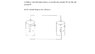# Batteries in circuit, terminal voltage

• scholio

## Homework Statement

two batteries are connected as shown, see attached 'drawing.' what is the terminal voltage across each battery

## Homework Equations

electric potential V = IR where I is current, R is resistance

I = V_0/R * e^(-t/RC) where t is time, C is capacitance <--RC circuit, discharging (10V batt.)

I = dQ/dt where t is time, Q is charge

I = epsilon/R e^(-t/RC) where epsilon is emf <---RC circuit, charging (5V battery)

## The Attempt at a Solution

i am not too sure about how to approach the problem but by looking at the drawing, i am making the assumption that the current follows a counterclockwise path and that the 10V battery is discharging -->doing work on the 5V battery?

is my assumption correct, if not, how should i approach the problem?

help appreciated

#### Attachments

•batt.JPG
10.3 KB · Views: 456